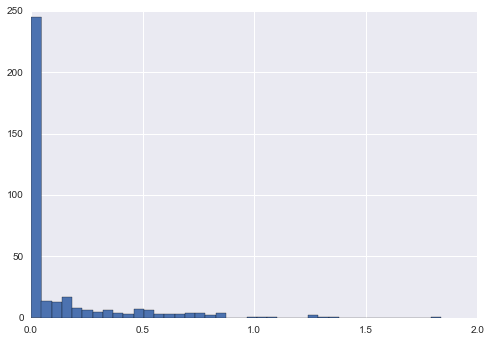## 9.8 比较，掩码和布尔逻辑

### 示例：统计雨天

``````import numpy as np
import pandas as pd

# 使用 pandas 将降雨量英寸提取为 NumPy 数组
inches = rainfall / 254  # 1/10mm -> 英寸
inches.shape

# (365,)
``````

``````%matplotlib inline
import matplotlib.pyplot as plt
import seaborn; seaborn.set()  # 设置绘图风格

plt.hist(inches, 40);
``````### 作为`ufunc`的比较运算

NumPy 还将比较运算符，例如`<`（小于）和`>`（大于），实现为逐元素的`ufunc`。这些比较运算符的结果始终是布尔数据类型的数组。所有六种标准比较操作都可用：

``````x = np.array([1, 2, 3, 4, 5])

x < 3  # 小于

# array([ True,  True, False, False, False], dtype=bool)

x > 3  # 大于

# array([False, False, False,  True,  True], dtype=bool)

x <= 3  # 小于等于

# array([ True,  True,  True, False, False], dtype=bool)

x >= 3  # 大于等于

# array([False, False,  True,  True,  True], dtype=bool)

x != 3  # 不等于

# array([ True,  True, False,  True,  True], dtype=bool)

x == 3  # 等于

# array([False, False,  True, False, False], dtype=bool)
``````

``````(2 * x) == (x ** 2)

# array([False,  True, False, False, False], dtype=bool)
``````

`==` `np.equal` `!=` `np.not_equal`
`<` `np.less` `<=` `np.less_equal`
`>` `np.greater` `>=` `np.greater_equal`

``````rng = np.random.RandomState(0)
x = rng.randint(10, size=(3, 4))
x

'''
array([[5, 0, 3, 3],
[7, 9, 3, 5],
[2, 4, 7, 6]])
'''

x < 6
'''
array([[ True,  True,  True,  True],
[False, False,  True,  True],
[ True,  True, False, False]], dtype=bool)
'''
``````

### 使用布尔数组

``````print(x)

'''
[[5 0 3 3]
[7 9 3 5]
[2 4 7 6]]
'''
``````

#### 对元素计数

``````# 多少个值小于 6
np.count_nonzero(x < 6)

# 8
``````

``````np.sum(x < 6)

# 8
``````

`sum()``的好处就是和其他NumPy聚合函数一样，这个求和也可以沿着行或列来完成：

``````# 每一行有多少个值小于 6
np.sum(x < 6, axis=1)

# array([4, 2, 2])
``````

``````# 存在大于 8 的值吗？
np.any(x > 8)

# True

# 存在小于 0 的值吗？
np.any(x < 0)

# False

# 所有值都小于 10 吗？
np.all(x < 10)

# True

# 所有值都等于 6 吗？
np.all(x == 6)

# False
``````

`np.all``np.any`也可用于特定的轴。例如：

``````# 每一行的所有值都小于 4 吗？
np.all(x < 8, axis=1)

# array([ True, False,  True], dtype=bool)
``````

#### 布尔运算符

``````np.sum((inches > 0.5) & (inches < 1))

# 29
``````

``````inches > (0.5 & inches) < 1
``````

``````np.sum(~( (inches <= 0.5) | (inches >= 1) ))

# 29
``````

`&` `np.bitwise_and` `|` `np.bitwise_or`
`^` `np.bitwise_xor` `~` `np.bitwise_not`

``````print("Number days without rain:      ", np.sum(inches == 0))
print("Number days with rain:         ", np.sum(inches != 0))
print("Days with more than 0.5 inches:", np.sum(inches > 0.5))
print("Rainy days with < 0.2 inches  :", np.sum((inches > 0) &
(inches < 0.2)))

'''
Number days without rain:       215
Number days with rain:          150
Days with more than 0.5 inches: 37
Rainy days with < 0.2 inches  : 75
'''
``````

### 作为掩码的布尔数组

``````x

'''
array([[5, 0, 3, 3],
[7, 9, 3, 5],
[2, 4, 7, 6]])
'''
``````

``````x < 5

'''
array([[False,  True,  True,  True],
[False, False,  True, False],
[ True,  True, False, False]], dtype=bool)
'''
``````

``````x[x < 5]

# array([0, 3, 3, 3, 2, 4])
``````

``````# 为所有雨天构造掩码
rainy = (inches > 0)

# 为所有夏天构造掩码（6 月 21 日是第 172 天）
days = np.arange(365)
summer = (days > 172) & (days < 262)

print("Median precip on rainy days in 2014 (inches):   ",
np.median(inches[rainy]))
print("Median precip on summer days in 2014 (inches):  ",
np.median(inches[summer]))
print("Maximum precip on summer days in 2014 (inches): ",
np.max(inches[summer]))
print("Median precip on non-summer rainy days (inches):",
np.median(inches[rainy & ~summer]))

'''
Median precip on rainy days in 2014 (inches):    0.194881889764
Median precip on summer days in 2014 (inches):   0.0
Maximum precip on summer days in 2014 (inches):  0.850393700787
Median precip on non-summer rainy days (inches): 0.200787401575
'''
``````

### 注：使用关键字`and/or`与运算符`&/|`

``````bool(42), bool(0)

# (True, False)

bool(42 and 0)

# False

bool(42 or 0)

# True
``````

``````bin(42)

# '0b101010'

bin(59)

# '0b111011'

bin(42 & 59)

# '0b101010'

bin(42 | 59)

# '0b111011'
``````

``````A = np.array([1, 0, 1, 0, 1, 0], dtype=bool)
B = np.array([1, 1, 1, 0, 1, 1], dtype=bool)
A | B

# array([ True,  True,  True, False,  True,  True], dtype=bool)
``````

``````A or B

'''
---------------------------------------------------------------------------

ValueError                                Traceback (most recent call last)

<ipython-input-38-5d8e4f2e21c0> in <module>()
----> 1 A or B

ValueError: The truth value of an array with more than one element is ambiguous. Use a.any() or a.all()
'''
``````

``````x = np.arange(10)
(x > 4) & (x < 8)

# array([False, False, False, False, False,  True,  True,  True, False, False], dtype=bool)
``````

``````(x > 4) and (x < 8)

'''
---------------------------------------------------------------------------

ValueError                                Traceback (most recent call last)

<ipython-input-40-3d24f1ffd63d> in <module>()
----> 1 (x > 4) and (x < 8)

ValueError: The truth value of an array with more than one element is ambiguous. Use a.any() or a.all()
'''
``````